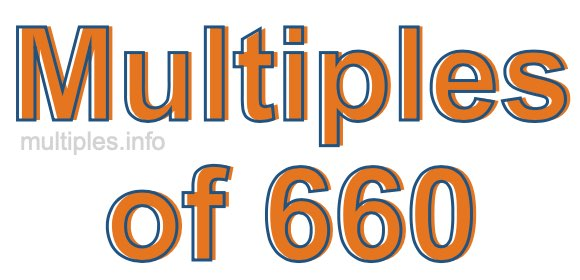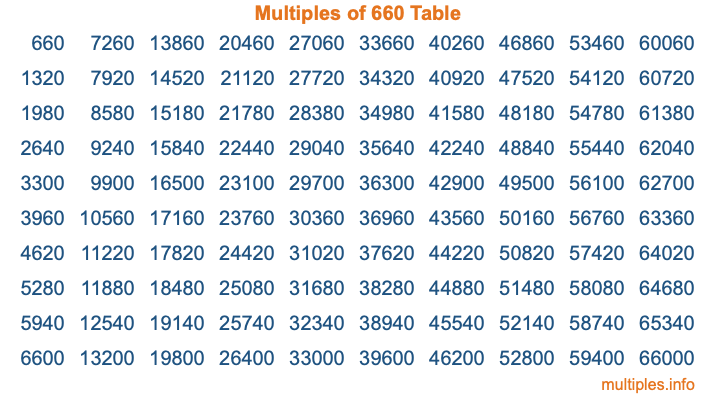Multiples of 660Welcome to the Multiples of 660 page. Here we will first teach you everything you will ever need to know about the multiples of 660, and then give you a study guide summary of everything we taught you to make sure you remember it all. Use this page to look up facts and learn information about the multiples of 660. This page will make you a multiples of six hundred sixty expert!

Definition of Multiples of 660
Multiples of 660 are all the numbers that when divided by 660 equal an integer. Each of the multiples of 660 are called a multiple. A multiple of 660 is created by multiplying 660 by an integer.

Therefore, to create a list of multiples of 660, you start with 1 multiplied by 660, then 2 multiplied by 660, then 3 multiplied by 660, and so on for as long as you want. Thus, the list of the first five multiples of 660 is 660, 1320, 1980, 2640, and 3300. To see a larger list of multiples of 660, see the printable image of Multiples of 660 further down on this page. We also have a category where you can choose any nth multiple of 660.

Multiples of 660 Checker
The Multiples of 660 Checker below checks to see if any number of your choice is a multiple of 660. In other words, it checks to see if there is any number (integer) that when multiplied by 660 will equal your number. To do that, we divide your number by 660. If the the quotient is an integer, then your number is a multiple of 660.

Is  a multiple of 660?

Least Common Multiple of 660 and ...
A Least Common Multiple (LCM) is the lowest multiple that two or more numbers have in common. This is also called the smallest common multiple or lowest common multiple and is useful to know when you are adding our subtracting fractions. Enter one or more numbers below (660 is already entered) to find the LCM.

Check out our LCM Calculator if you need more details about the Least Common Multiple or if you need the LCM for different numbers for adding and subtraction fractions.

nth Multiple of 660
As we stated above, 660 is the first multiple of 660, 1320 is the second multiple of 660, 1980 is the third multiple of 660, and so on. Enter a number below to find the nth multiple of 660.

th multiple of 660

Multiples of 660 vs Factors of 660
660 is a multiple of 660 and a factor of 660, but that is where the similarities end. All postive multiples of 660 are 660 or greater than 660. All positive factors of 660 are 660 or less than 660.

Below is the beginning list of multiples of 660 and the factors of 660 so you can compare:

Multiples of 660: 660, 1320, 1980, 2640, 3300, etc.

Factors of 660: 1, 2, 3, 4, 5, 6, 10, 11, 12, 15, 20, 22, 30, 33, 44, 55, 60, 66, 110, 132, 165, 220, 330, 660

As you can see, the multiples of 660 are all the numbers that you can divide by 660 to get a whole number. The factors of 660, on the other hand, are all the whole numbers that you can multiply by another whole number to get 660.

It's also interesting to note that if a number (x) is a factor of 660, then 660 will also be a multiple of that number (x).

Multiples of 660 vs Divisors of 660
The divisors of 660 are all the integers that 660 can be divided by evenly. Below is a list of the divisors of 660.

Divisors of 660: 1, 2, 3, 4, 5, 6, 10, 11, 12, 15, 20, 22, 30, 33, 44, 55, 60, 66, 110, 132, 165, 220, 330, 660

The interesting thing to note here is that if you take any multiple of 660 and divide it by a divisor of 660, you will see that the quotient is an integer.

Multiples of 660 Table
Below is an image of the first 100 multiples of 660 in a table. The table is in chronological order, column by column. The first column has the first ten multiples of 660, the second column has the next ten multiples of 660, and so on.The Multiples of 660 Table is also referred to as the 660 Times Table or Times Table of 660. You are welcome to print out our table for your studies.

Negative Multiples of 660
Although not often discussed or needed in math, it is worth mentioning that you can make a list of negative multiples of 660 by multiplying 660 by -1, then by -2, then by -3, and so on, to get the following list of negative multiples of 660:

-660, -1320, -1980, -2640, -3300, etc.

Multiples of 660 Summary
Below is a summary of important Multiples of 660 facts that we have discussed on this page. To retain the knowledge on this page, we recommend that you read through the summary and explain to yourself or a study partner why they hold true.

There are an infinite number of multiples of 660.

A multiple of 660 divided by 660 will equal a whole number.

660 divided by a factor of 660 equals a divisor of 660.

The nth multiple of 660 is n times 660.

The largest factor of 660 is equal to the first positive multiple of 660.

660 is a multiple of every factor of 660.

660 is a multiple of 660.

A multiple of 660 divided by a divisor of 660 equals an integer.

660 divided by a divisor of 660 equals a factor of 660.

Any integer times 660 will equal a multiple of 660.

Multiples of a Number
Here you can get the multiples of another number, all with the same attention to detail as we did for multiples of 660 on this page.

Multiples of
Multiples of 661
Did you find our page about multiples of six hundred sixty educational? Do you want more knowledge? Check out the multiples of the next number on our list!